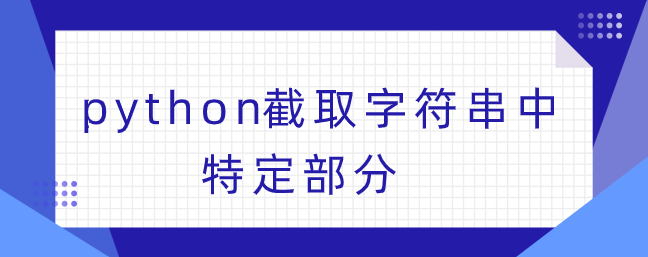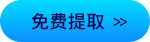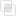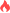python截取字符串中特定部分

Python 字符串2021-04-25 10:19:43523浏览 · 0收藏 · 0评论1、截取特定长度的字符串。使用s[ : ]，截取字符串中一段字符，遵循左闭右开原则，从0开始，到X-1结束。

```s = "abcdefgh"

#1、a range of characters   取一段字符
print(s[0:3])               #abc
print(s[1:3])               #bc
print("-----------")
print(s[2:3])               #c
print(s[3:3])               #None

#2、with default parameters 缺省参数
print(s[3:])                #defgh
print(s[:3])                #abc
print(s[:])                 #abcdefgh
print("-----------")

#3、with a step parameter   步长
print("This is not as common, but perfectly ok.")
print(s[1:7:2])             #bdf    2是步长，即输出1、1+2、1+2+2 （1+2+2+2=7超出范围）
print(s[1:7:3])             #be     3是步长，即输出1、1+3  （1+3+3=7超出范围）```

2、根据指定的字符截取字符串，首先获得字符的下标记位置。

Python提供index函数，检查字符串是否包含子字符串，通常表现为特定字符、特定字符。

```str1 = "Hello.python";

str2 = ".";

print str1.index(str2);#结果5

print str1.index(str2, 2);#结果5

print str1.index(str2, 10);#结果报错，没找到子字符串

str2 = ".";

print str1.index(str2);#结果5

print str1[:str1.index(str2)] #获取 "."之前的字符(不包含点) 结果 Hello

print str1[str1.index(str2):] ; #获取 "."之前的字符(包含点) 结果.python```python查询字符串参数python打印字符串的方法python字符串有几种常见方法Chapter Three : Python 序列之字符串操作详解python字符串常用方法有哪些python字符串如何取值python字符串格式化的方法整理python字符串如何简单运算php数组转json字符串python使用f格式化字符串python中str()函数转换字符串python str.zfill填充字符串python使用f.read()返回字符串php数组转字符串java循环和递归的区别309

java枚举如何实现单例372

java中final域的内存语义理解705